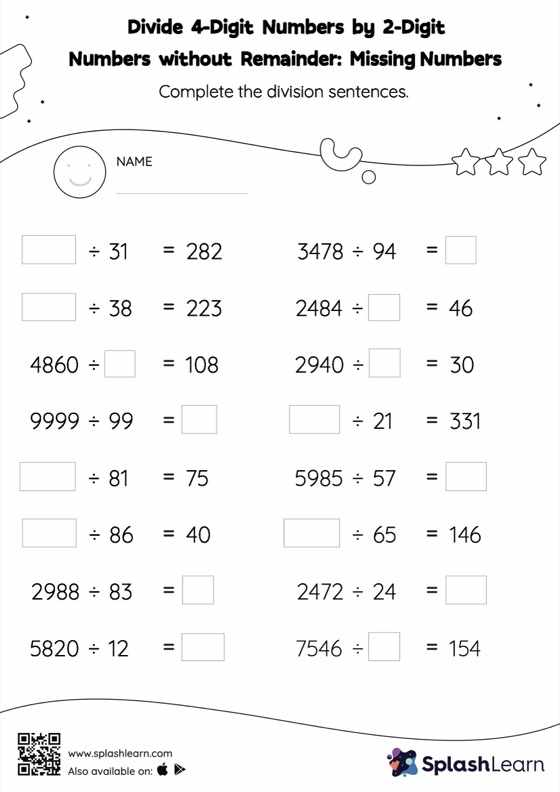# Divide 4-Digit Numbers by 2-Digit Numbers without Remainder: Missing Numbers Worksheet

Home > Divide 4-Digit Numbers by 2-Digit Numbers without Remainder: Missing NumbersGive your little one the opportunity to dive deeper into division with this divide 4-digit numbers by 2-digit numbers without remainder worksheet. In divide 4-digit numbers by 2-digit numbers without remainder worksheet, students find the missing number using their understanding of division facts and the long division method. No remainders exist for this set of problems.